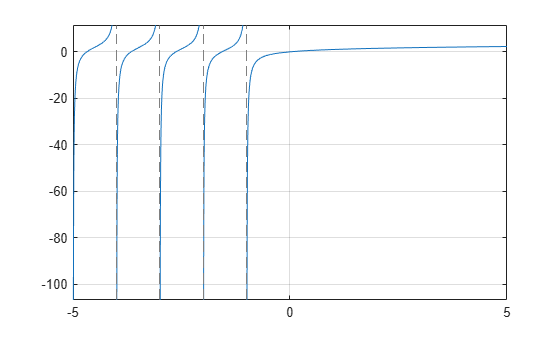# harmonic

Harmonic function (harmonic number)

## Syntax

``harmonic(x)``

## Description

example

````harmonic(x)` returns the harmonic function of `x`. For integer values of `x`, `harmonic(x)` generates harmonic numbers.```

## Examples

### Generate Harmonic Numbers

Generate the first 10 harmonic numbers.

`harmonic(sym(1:10))`
```ans = [ 1, 3/2, 11/6, 25/12, 137/60, 49/20, 363/140, 761/280, 7129/2520, 7381/2520]```

### Harmonic Function for Numeric and Symbolic Arguments

Find the harmonic function for these numbers. Since these are not symbolic objects, you get floating-point results.

`harmonic([2 i 13/3])`
```ans = 1.5000 + 0.0000i 0.6719 + 1.0767i 2.1545 + 0.0000i```

Find the harmonic function symbolically by converting the numbers to symbolic objects.

`y = harmonic(sym([2 i 13/3]))`
```y = [ 3/2, harmonic(1i), 8571/1820 - (pi*3^(1/2))/6 - (3*log(3))/2]```

If the denominator of `x` is 2, 3, 4, or 6, and |x| < 500, then the result is expressed in terms of `pi` and `log`.

Use `vpa` to approximate the results obtained.

`vpa(y)`
```ans = [ 1.5, 0.67186598552400983787839057280431... + 1.07667404746858117413405079475i,... 2.1545225442213858782694336751358]```

For |x| > 1000, `harmonic` returns the function call as it is. Use `vpa` to force `harmonic` to evaluate the function call.

```harmonic(sym(1001)) vpa(harmonic(sym(1001)))```
```ans = harmonic(1001) ans = 7.4864698615493459116575172053329```

### Harmonic Function for Special Values

Find the harmonic function for special values.

`harmonic([0 1 -1 Inf -Inf])`
```ans = 0 1 Inf Inf NaN```

### Harmonic Function for Symbolic Functions

Find the harmonic function for the symbolic function `f`.

```syms f(x) f(x) = exp(x) + tan(x); y = harmonic(f)```
```y(x) = harmonic(exp(x) + tan(x))```

### Harmonic Function for Symbolic Vectors and Matrices

Find the harmonic function for elements of vector `V` and matrix `M`.

```syms x V = [x sin(x) 3*i]; M = [exp(i*x) 2; -6 Inf]; harmonic(V) harmonic(M) ```
```ans = [ harmonic(x), harmonic(sin(x)), harmonic(3i)] ans = [ harmonic(exp(x*1i)), 3/2] [ Inf, Inf]```

### Plot Harmonic Function

Plot the harmonic function from `x` = -5 to `x` = 5.

```syms x fplot(harmonic(x),[-5 5]) grid on```### Differentiate and Find Limit of Harmonic Function

The functions `diff` and `limit` handle expressions containing `harmonic`.

Find the second derivative of `harmonic(x^2+1)`.

```syms x diff(harmonic(x^2+1),x,2)```
```ans = 2*psi(1, x^2 + 2) + 4*x^2*psi(2, x^2 + 2)```

Find the limit of `harmonic(x)` as `x` tends to ∞ and of `(x+1)*harmonic(x)` as `x` tends to -1.

```syms x limit(harmonic(x),Inf) limit((x+1)*harmonic(x),-1)```
```ans = Inf ans = -1```

### Taylor Series Expansion of Harmonic Function

Use `taylor` to expand the harmonic function in terms of the Taylor series.

```syms x taylor(harmonic(x))```
```ans = (pi^6*x^5)/945 - zeta(5)*x^4 + (pi^4*x^3)/90... - zeta(3)*x^2 + (pi^2*x)/6 ```

### Expand Harmonic Function

Use `expand` to expand the harmonic function.

```syms x expand(harmonic(2*x+3))```
```ans = harmonic(x + 1/2)/2 + log(2) + harmonic(x)/2 - 1/(2*(x + 1/2))... + 1/(2*x + 1) + 1/(2*x + 2) + 1/(2*x + 3) ```

## Input Arguments

collapse all

Input, specified as number, vector, matrix, or as a multidimensional array or symbolic variable, expression, function, vector, matrix, or multidimensional array.

collapse all

### Harmonic Function

The harmonic function for x is defined as

`$\mathrm{harmonic}\left(x\right)=\underset{k=1}{\overset{x}{\Sigma }}\frac{1}{k}$`

It is also defined as

`$\mathrm{harmonic}\left(x\right)=\Psi \left(x+1\right)+\gamma$`

where Ψ(x) is the polygamma function and γ is the Euler-Mascheroni constant.

## Algorithms

The harmonic function is defined for all complex arguments z except for negative integers -1, -2,... where a singularity occurs.

If `x` has denominator 1, 2, 3, 4, or 6, then an explicit result is computed and returned. For other rational numbers, `harmonic` uses the functional equation $\mathrm{harmonic}\left(x+1\right)=\mathrm{harmonic}\left(x\right)+\frac{1}{x}$ to obtain a result with an argument x from the interval [0, 1].

`expand` expands `harmonic` using the equations $\mathrm{harmonic}\left(x+1\right)=\mathrm{harmonic}\left(x\right)+\frac{1}{x}$, $\mathrm{harmonic}\left(-x\right)=\mathrm{harmonic}\left(x\right)-\frac{1}{x}+\pi \mathrm{cot}\left(\pi x\right)$, and the Gauss multiplication formula for `harmonic(kx)`, where k is an integer.

`harmonic` implements the following explicit formulae:

`$\mathrm{harmonic}\left(\frac{-1}{2}\right)=-2\mathrm{ln}\left(2\right)$`

`$\mathrm{harmonic}\left(-\frac{2}{3}\right)=-\frac{3}{2}\mathrm{ln}\left(3\right)-\frac{\sqrt{3}}{6}\pi$`

`$\mathrm{harmonic}\left(-\frac{1}{3}\right)=-\frac{3}{2}\mathrm{ln}\left(3\right)+\frac{\sqrt{3}}{6}\pi$`

`$\mathrm{harmonic}\left(\frac{-3}{4}\right)=-3\mathrm{ln}\left(2\right)-\frac{\pi }{2}$`

`$\mathrm{harmonic}\left(\frac{-1}{4}\right)=-3\mathrm{ln}\left(2\right)+\frac{\pi }{2}$`

`$\mathrm{harmonic}\left(\frac{-5}{6}\right)=-2\mathrm{ln}\left(2\right)-\frac{3}{2}\mathrm{ln}\left(3\right)-\frac{\sqrt{3}}{2}\pi$`

`$\mathrm{harmonic}\left(\frac{-1}{6}\right)=-2\mathrm{ln}\left(2\right)-\frac{3}{2}\mathrm{ln}\left(3\right)+\frac{\sqrt{3}}{2}\pi$`

`$\mathrm{harmonic}\left(0\right)=0$`

`$\mathrm{harmonic}\left(\frac{1}{2}\right)=2-2\mathrm{ln}\left(2\right)$`

`$\mathrm{harmonic}\left(\frac{1}{3}\right)=3-\frac{3}{2}\mathrm{ln}\left(3\right)-\frac{\sqrt{3}}{6}\pi$`

`$\mathrm{harmonic}\left(\frac{2}{3}\right)=\frac{3}{2}-\frac{3}{2}\mathrm{ln}\left(3\right)+\frac{\sqrt{3}}{6}\pi$`

`$\mathrm{harmonic}\left(\frac{1}{4}\right)=4-3\mathrm{ln}\left(2\right)-\frac{\pi }{2}$`

`$\mathrm{harmonic}\left(\frac{3}{4}\right)=\frac{4}{3}-3\mathrm{ln}\left(2\right)+\frac{\pi }{2}$`

`$\mathrm{harmonic}\left(\frac{1}{6}\right)=6-2\mathrm{ln}\left(2\right)-\frac{3}{2}\mathrm{ln}\left(3\right)-\frac{\sqrt{3}}{2}\pi$`

`$\mathrm{harmonic}\left(\frac{5}{6}\right)=\frac{6}{5}-2\mathrm{ln}\left(2\right)-\frac{3}{2}\mathrm{ln}\left(3\right)+\frac{\sqrt{3}}{2}\pi$`

`$\mathrm{harmonic}\left(1\right)=1$`

`$\mathrm{harmonic}\left(\infty \right)=\infty$`

`$\mathrm{harmonic}\left(-\infty \right)=NaN$`

## Version History

Introduced in R2014a